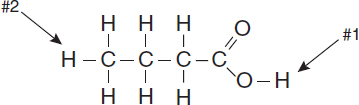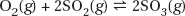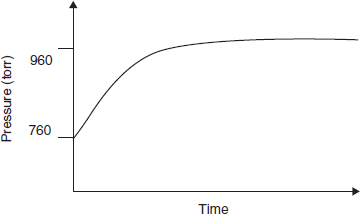# AP Chemistry Practice Test 34

### Test Information12 questions18 minutes

1. S(s) + O2(g) → SO2(g)

The reaction between sulfur and oxygen, written in equation form above, can be interpreted in all of the following ways except

`Questions 2-3 refer to the following information.`

2. Which hydrogen in butanoic acid, shown above, will ionize and why?

3. Butanoic acid C4H8O2, shown above, gives rancid butter its foul smell. It has a Ka = 1.5 × 10-5. If a 0.0100 M solution of butanoic acid is prepared, the expected pH will be in which one of the following pH ranges?

`Questions 4-6 refer to the following information.Tl+(aq) + Cl-(aq) → TlCl(s)A chemist mixes a dilute solution of thallium perchlorate with a dilute solution of potassium chloride to precipitate thallium chloride (Ksp = 1.9 × 10-4).`

4. Which of the following is a molecular equation for the reaction described above?

5. If equal volumes of 2.0 millimolar solutions are mixed, which of the following particulate views represents the experiment after the reactants are mixed thoroughly and solids are given time to precipitate?

6. Within a factor of 10, what is the approximate molar solubility of thallium chloride in distilled water?

`Questions 7-12 refer to the following information.SO2(g) is produced in the combustion of coal, oil, gasoline and many other natural products. In the atmosphere, it reacts with oxygen to form SO3(g) via the equation above. A pure sample of SO3(g) is placed into a rigid, evacuated 0.500 L container. The initial pressure of the SO3(g) is 760 atm. The temperature is held constant until the system reaches equilibrium. The figure below shows how the pressure of the system changes in reaching equilibrium.`

7. Why does the pressure rise in this experiment?

8. The pressure versus time curve can be used to

9. What will the final pressure be if this reaction goes to completion?

10. Sulfur trioxide decomposed to form some sulfur dioxide and molecular oxygen. What is the proper form for the equilibrium expression for the reaction that actually occurred?

11. Which diagram is an appropriate description of the system if more SO2(g) is rapidly injected, at time = 0, into the container after equilibrium is established?

12. Why are SO2(g) and SO3(g) pollutants to be concerned about?